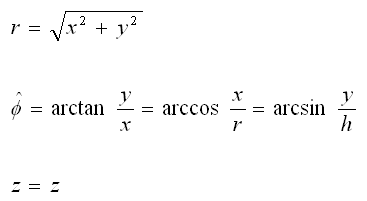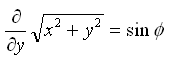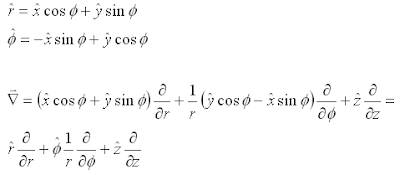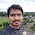Cool Math Tricks: Deriving the Divergence, (Del or Nabla) into New (Cylindrical) Coordinate Systems

The following is a pretty lengthy procedure, but converting the divergence, (nabla, del) operator between coordinate systems comes up pretty often. While there are tables for converting between common coordinate systems, there seem to be fewer explanations of the procedure for deriving the conversion, so here goes!

What do we actually want?

To convert the Cartesian nablato the nabla for another coordinate system, say… cylindrical coordinates.What we’ll need:

1. The Cartesian Nabla:2. A set of equations relating the Cartesian coordinates to cylindrical coordinates:3. A set of equations relating the Cartesian basis vectors to the basis vectors of the new coordinate system:How to do it:

Use the chain rule for differentiation to convert the derivatives with respect to the Cartesian variables to derivatives with respect to the cylindrical variables.

The chain rule can be used to convert a differential operator in terms of one variable into a series of differential operators in terms of other variables that the first variable depends on. This is exactly what we need because we want to start out with a differential operator in terms of x,in our Cartesian to cylindrical example and convert it to differential operators in terms of the cylindrical variables, . For x, the chain rule gives us:NOTE: More generally, if you’re given a differential operator in terms of a variable, u, that can be expressed in terms of n other variables, v, then to convert the differential operator in terms of u to differential operators in terms of the v’s the chain rule gives:This is explained very nicely and in more detail here and here.

OK, so let’s get to work converting the three differential operators of the Cartesian nabla into differential operators in terms of cylindrical coordinates. We start with x,using,We can construct the first differential term above as:but, we really need to get all the x’s and y’s out of the mix and just have cylindrical coordinates remaining. So, using a few substitutions we get:Now, follow the same procedure for the next differential term in the chain rule.Remember though that r depends on x and y, so when we differentiate, we need to expand r and use the simple one-variable chain rule. Using a table of derivates for the arccosine, we get:The final derivative is taken using the quotient rule:We already know from our work above thatsoNow, we start the lengthy process of simplifying:Because z is the same z as in the Cartesian system, it doesn’t depend on x, (exactly as in the Cartesian system), so we haveand our complete conversion for the x derivative term is:Now use the same techniques to convert the differential for y:Starting in a similar manner:Then, evaluating the second term of the chain rule we get:Remember again that r depends on x and y, so when we differentiate, we need to expand r and use the one variable chain rule.The final derivative is taken using the quotient rule:We already know from our work above thatsoNow, we simplify again:So,Finally, since z is not transformed between coordinate systems:Now that we have each differential converted, we can write the nabla operator asWhich is kind of a mess! We know that when we’re finished we expect to see nicely grouped cylindrical differential terms with cylindrical unit vectors, so let’s group on the cylindrical differentialsNow remembering our original definition of cylindrical unit vectors,We can make two more substitutions and arrive at the final result for nabla in cylindrical coordinates!Anonymous said…
Hi Hamilton,

very nice tutorial. Following your reasoning I was able to derive the Nabla from Cartesian coordinate system to my own defined non-standard spherical coordinate system. And it worked perfectly!

Thanks again.

HernanAnonymous said…
Thank you....

great help!

--SuJohn F. said…
Hi,
Good article. I noticed a shortcut for d phi / dx and d phi / dy. If you use phi = arctan(y/x),
d phi / dx = 1/(1+(y/x)^2).d/dx(y/x)
=-1/(1+(y/x)^2).y/x^2
=-y/(x^2+y^2)
=-sin(phi)/r
similar for d phi/dy, and avoids a lot of messy algebra.Anonymous said…Anonymous said…
Firstly, thanks for this, I've been trying in vain to derive something similar and I've finally noticed that I've been using the wrong transformation of unit vectors!

Similarly to previous comments, this particular result falls out fairly quickly when you note that r_x = cos(phi); r_y = sin(phi); phi_x = -sin(phi)/r and phi_y=cos(phi)/r. Otherwise a good exercise in the chain rule!mani said…
hi,
i'm from india and i found your explanation extremely helpful.i was able to derive expressions for divergence and curl on my own by following a method similar to yours.
it was enlightening.
thanks.Doughboy said…
Your derivation is great, but it's too lengthy. You can actually derive it starting with the definition of divergence, which is the limit as the volume of a closed surface approaches zero of the flux through that surface. Taking the surface as the standard differential volume unit in cylindrical coordinates, you can directly derive it much more quickly than going through the chain rule and coordinate transformations.Hamilton said…
Thank you Doughboy! That's an awesome pointer! It took me awhile to work through all the details of what you said, but in the end it's far easier and left me with a physical understanding of the derivation instead of just a mechanical list of steps. Expect to see a post on deriving the spherical laplacian in this way soon. Thanks again!Hamilton said…
This comment has been removed by the author.Hamilton said…
Hi Doughboy,
Is the following for spherical coordinates about what you had in mind?

Thanks again! You made my homework this week a lot easier and much more of a learning experience, (in a good way).Unknown said…
Thanks a lot. I was searching for this material and I found great your explanation !!Thanks a lot. I was searching for this material and I found great your explanation !!Anonymous said…
Awesome stuff man!
Your work saved me a lot of time
Thanks a lotAnonymous said…
thanxs bro.. great helpAnonymous said…
Thank you a lot man!!!

The Valentine's Day Magnetic Monopole

There's an assymetry to the form of the two Maxwell's equations shown in picture 1.  While the divergence of the electric field is proportional to the electric charge density at a given point, the divergence of the magnetic field is equal to zero.  This is typically explained in the following way.  While we know that electrons, the fundamental electric charge carriers exist, evidence seems to indicate that magnetic monopoles, the particles that would carry magnetic 'charge', either don't exist, or, the energies required to create them are so high that they are exceedingly rare.  That doesn't stop us from looking for them though!

Keeping with the theme of Fairbank and his academic progeny over the semester break, today's post is about the discovery of a magnetic monopole candidate event by one of the Fairbank's graduate students, Blas Cabrera.  Cabrera was utilizing a loop type of magnetic monopole detector.  Its operation is in concept very simpl…

Kids R Kapable

Just a little note to concerned ‘grownups’ everywhere.  If you look at a kid—and I mean really look—I don’t mean notice a person shorter than you, I mean make eye contact, notice their facial expression and observe their body language—If you look at a kid, don’t assume they need your help unless they’re obviously distressed, or ask for it.  You might think this is difficult call to make.  You might think, not having kids of your own, that you’re unable to make this determination.  You are.  You do in fact, already have the skills even if you’ve never been around kids  It’s a remarkably simple call to make, just use the exact same criteria you would for determining if an adult was in distress.  Because, guess what, kids and adults are in fact the same species of animal and communicate in the same way.  Honest.  If someone—adult or child—doesn’t need your help, feel free to say hello, give a wave, give a smile, but don’t—do not—try to force help on anyone that doesn’t want or need it.

Y…